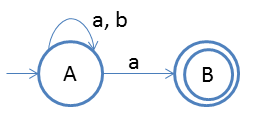# Introduction to NFA

NFA means non-deterministic finite automata.
The main difference between NFA and DFA is that we can move to > 1 states on single input.

We basically use NFA in backtracking and exhaustive search.

Following are the parameters of NFA:
SymbolDescription
QSet of finite states
ΣInput alphabet
q0Start State
δTransition Function: δ : Q X Σ -> 2Q
FFinal State

Now we will understand using one example:

#### End with 'a'

L = {a, aa, ab, ...}Note: 1 Every DFA is NFA but vice-versa is not true.
And the above property is true because the δ of DFA is ⊆ δ of DFA
As δ : Q X Σ -> 2Q ⊇ Q X Σ -> Q
Note 2: We do not have dead states in NFA
Note 3: NFA and DFA both are equivalant in power (This is because "Every DFA is NFA" and "We can convert NFA to DFA")